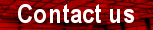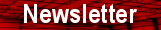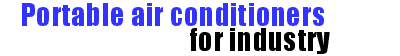Coolingspacer
 Search this site: Using :  All Words  Any Words PhraseW. Tombling Ltd.

Wembley House
Dozens Bank
West Pinchbeck
Spalding
Lincolnshire
PE11 3ND
U.K.You are here:- home > cooling index > air conditioning index > determining the size of air conditioner required

# Heat load or heat gain

A building or room gains heat from many sources. Inside occupants, computers, copiers, machinery, and lighting all produce heat. Warm air from outside enters through open doors and windows, or as ‘leakage’ though the structure. However the biggest source of heat is solar radiation from the sun, beating down on the roof and walls, and pouring through the windows, heating internal surfaces.

The sum of all these heat sources is know as the heat gain (or heat load) of the building, and is expressed either in BTU (British Thermal Units) or Kw (Kilowatts).

For an air conditioner to cool a room or building its output must be greater than the heat gain. It is important before purchasing an air conditioner that a heat load calculation is performed to ensure it is big enough for the intended application.

There are several different methods of calculating the heat load for a given area:

### Quick calculation for offices

For offices with average insulation and lighting, 2/3 occupants and 3/4 personal computers and a photocopier, the following calculations will suffice:
Heat load (BTU) = Length (ft.) x Width (ft.) x Height (ft.) x 4

Heat load (BTU) = Length (m) x Width (m) x Height (m) x 141

If there are any additional significant sources of heat, for instance floor to ceiling south facing windows, or equipment that produces lots of heat, the above method will underestimate the heat load. In which case the following method should be used instead.

### A more accurate heat load calculation for any type of room or building

The heat gain of a room or building depends on:
The size of the area being cooled
The size and position of windows, and whether they have shading
The number of occupants
Heat generated by equipment and machinery
Heat generated by lighting
By calculating the heat gain from each individual item and adding them together, an accurate heat load figure can be determined.

Step One

Calculate the area in square feet of the space to be cooled, and multiply by 31.25
Area BTU = length (ft.) x width (ft.) x 31.25

Step Two

Calculate the heat gain through the windows. If the windows don’t have shading multiply the result by 1.4
North window BTU = Area of North facing windows (m. sq.) x 164

If no shading, North window BTU = North window BTU x 1.4

South window BTU = Area of South facing windows (m. sq.) x 868

If no shading, South window BTU = South window BTU x 1.4
Total window BTU = North window + South window

Step Three

Calculate the heat generated by occupants, allow 600 BTU per person.
Occupant BTU = number of people x 600

Step Four

Calculate the heat generated by each item of machinery - copiers, computers, ovens etc. Find the power in watts for each item, add them together and multiply by 3.4
Equipment BTU = total equipment watts x 3.4

Step Five

Calculate the heat generated by lighting. Find the total wattage for all lighting and multiply by 4.25

Lighting BTU = total lighting watts x 4.25

Step Six

Total heat load BTU = Area BTU + Total Window BTU + Occupant BTU + Equipment BTU + Lighting BTU

Step Seven

Divide the heat load by the cooling capacity of the air conditioning unit in BTU, to determine how many air conditioners are needed.
Number of a/c units required = Total heat load BTU / Cooling capacity BTU

### On line heat gain calculatorManually calculating the size of air conditioner required can appear to be a complicated task. To simplify the process we have created an on-line calculator, to access it click on the picture of a calculator opposite.

Disclaimer.
If you have any doubts about the size of air conditioner required you should contact a reputable air conditioning engineer.
The above methods of calculation are simplified; factors such as insulation levels and building construction have been ignored. The above should be considered as an approximate method of calculation only. W. Tombling Ltd. accepts no liability or claim arising from their use.

You are here:- home > cooling index > air conditioning index > determining the size of air conditioner required